# Numbers and Math Operations

## Measured vs. Exact Numbers

Exact numbers are numbers that are exact by definition, such as:

1 inch = 2.54 cm or 1 gallon = 231 cubic inches or 1 foot = 12 inches or 1 meter = 100 centimeters

or

numbers that come in integers and are not likely to be available in amounts smaller than integers.
(When you ask for seating in a restaurant, the number of people is an integer, an exact number.)

Measured numbers are an estimated amount, measured to a certain number of significant figures without the benefit of any natural unit or numbers that come from a mathematical operation such as averaging. You would get a measured number from using a meterstick to find the length of a board or using a graduated cylinder to find the volume of a liquid. Average numbers of people would make a measured number, even though people naturally come only in integers. The average family in the U.S. has 2.37 children.

## Scientific Notation

There are many very large and very small numbers in scientific studies. How would you like have to calculate with:

1 mol = 602,200,000,000,000,000,000,000 atoms

or

1 Dalton = 0.000,000,000,000,000,000,000,00165 g

You can streamline large or small numbers with scientific notation. The standard is that you place the decimal point after the first significant digit and adjust the exponent of ten so that there is no change in the value of the number. Think of the change as creating a new number with two parts, a digit part and an exponent part, from the old number. To put the decimal point behind the first digit, you must divide or multiply the original number by some integer power of ten. Then you must do the opposite (inverse) to the exponent part of the new expression so that there is no change in the value of the number.

(0.000,000,000,000,000,000,000,00165 x 1024) x 1/1024 = 1.65 x 10-24 or 1.65 E-24

(602,200,000,000,000,000,000,000/1023) x 1023 = 6.022 x 1023 or 6.022 E23

The original numbers have the same value as the exponential forms, but the exponential forms have the decimal point in the right place. The ‘right place’ is to the right of the first digit. The “E” in the number stands for exponent. Your scientific calculator will use the numbers in the shortened form, usually best represented by the “E” form. Don’t get caught making too much of this. You have seen it before. The number “five point two million” is the same as 5.2 E6. The number of the power of ten only indicates how many places you need to move the decimal to get the long form of the number back. The only question you might have trouble with is WHICH WAY to move the decimal. The easy way to remember that is: numbers that are less than one have negative exponent numbers in the scientific notation form, and numbers that are larger than one have positive exponent numbers. Very often chemistry professors will tell you they want answers in scientific notation if the number is larger than one thousand or smaller than one thousandth. Keep your professor happy. Find out exactly what is required in your course and follow the instructions to the letter.

The table below shows the fully written out number, the way we say the number in English, and the way to write the number in scientific notation. The numbers in scientific notation are to two significant digits.

 Full number Words for number Sci. notation styles 5,000,000,000 five billion (USA) 5.0 x 109     or     5.0 E9 500,000,000 five hundred million 5.0 x 108     or     5.0 E8 50,000,000 fifty million 5.0 x 107     or     5.0 E7 5,000,000 five million 5.0 x 106     or     5.0 E6 500,000 five hundred thousand 5.0 x 105     or     5.0 E5 50,000 fifty thousand 5.0 x 104     or     5.0 E4 5,000 five thousand 5.0 x 103     or     5.0 E3 500 five hundred 5.0 x 102     or     5.0 E2 50 fifty 5.0 x 101     or     5.0 E1 5 five 5.0 x 100     or     5.0 E0 0.5 five tenths 5.0 x 10-1     or     5.0 E-1 0.05 five hundredths 5.0 x 10-2     or     5.0 E-2 0.005 five thousandths 5.0 x 10-3     or     5.0 E-3 0.000,5 five ten-thousandths 5.0 x 10-4     or     5.0 E-4 0.000,05 five hundred-thousandths 5.0 x 10-5     or     5.0 E-5 0.000,005 five millionths 5.0 x 10-6     or     5.0 E-6 0.000,000,5 five ten-millionths 5.0 x 10-7     or     5.0 E-7 0.000,000,05 five hundred-millionths 5.0 x 10-8     or     5.0 E-8 0.000,000,005 five billionths 5.0 x 10-9     or     5.0 E-9

## Significance and Rounding

All the numbers in the above table have only two significant digits. Only the five and zero in each number has any numerical meaning other than place-holding. Let’s say that Delhi, India has five million people. We have expressed that number in one significant digit. That might be sufficient for such a number. Are we considering just people within the city limits? How about people who live outside the city and come in only for business? What year are we specifying? Let’s say we have enough information to number the population of Delhi at 5.1 million. That is now more a more accurate number that claims a two digit significance. What if we were to go absolutely wild and say that Delhi has 5.1376504 x 106 people? That is the same number, isn’t it? First, it ridiculously claims eight significant digits. Even worse, that calculates to 5,137,650.4 people, and people just don’t come in four-tenths of a person. Yes, you can claim to be far too accurate. With that in mind, here are the rules for considering the number of significant digits:

1. All non-zero digits are significant.

Every 1, 2, 3, 4, 5, 6, 7, 8, and 9 claims significance. The number, 123,456.789 has nine significant digits.

2. All leading and following zeros that are only place-holders are not significant.
These numbers have some magnitude-indicating zeros. The red (underlined) zeros are not significant in  4,200,000   (2 SF)  and   0.000,00.

3. All zeros between two other digits are significant.
The number 6.023 has a significant zero for a total of four significant digits.

4. All zeros to the right of the decimal and to the right of other digits are significant.
For instance, the number 43.500 has five significant digits, two of which are zeros.

You may hear the phrases significant digit, significant numeral, or significant figure to describe this idea. Chemtutor will sometimes shorten it to “sig figs,” or just “SF.”

We can round numbers to the proper number of significant digits by lopping off all digits past the number needed if less than five and rounding up the last needed digit if the following digit is five or more. For examples, here rounding to three significant digits:
3.4848 becomes 3.48;

4.1550 becomes 4.16;
5,786,899 becomes 5,790,000 (or 5.79 E6); and
0.000,347,00 becomes 0.000,347 (or 3.47 E-4).

How do you know when you need to round? In multiplication and division, the answer cannot have more significant digits than the number with the smallest number of significant digits used to calculate the answer. So a four significant digit number multiplied or divided by an eight significant digit number will result in a number that can only claim four significant digits. Wisconsin has 379 cities with about 5.1 thousand people in it. How many people live in all these small towns? 379 x 5.1 E3 = 1.9329 E6, but the answer can only claim two significant digits. The answer must be 1.9 E6 people because the number 5.1 E3 only has two significant digits.

How do you know where to round in actual measurements? The last digit is the one we get by estimation. For instance, if you have a graduated cylinder marked in milliliters and tenths of a milliliter, you should be able to estimate between the lines (interpolate) of tenths of milliliters and measure hundredths of milliliters.

The allowed significance works differently with adding and subtracting. The addition of a family of four to a city of three million does not significantly change the population of the city. If you have 1,578,000 chickens and you add 2,717 chickens to them, you have 1,581,000 chickens. Align these numbers one on top of the other so you can more easily see the reasoning behind this. No answer in subtraction or addition can have significant digits in COLUMNS in which on the right there is not a significant digit in each participant number. In the example, the columns with the zeros (in red) are not significant. The raw answer of 1,580,717 must be rounded to show that columns E, F, and G are not significant. The answer must then be rounded up because column E in the answer is greater than 5. The answer is 1,581,000 chickens.

 A B C D E F G 1 5 7 8 0 0 0 ______ _______ +_____ 2_____ 7_____ 1_____ 7_____ 1 5 8 0 7 1 7

It is just as important to know WHEN to round as HOW to round. In any math problem you should wait until the end to round; Only the final answer should be rounded. Carry as many significant digits as you can throughout the problem. On a calculator, the most efficient way to carry the maximum is to do all the calculation on the calculator. Arrange the problem so that you do not have to copy an intermediate answer only to re-enter it into the calculator. If you do find yourself needing to save numbers outside the calculator, copy several more significant digits than you think you need.

## Dimensional Analysis

Dimensional analysis is a system of using the units of quantities to guide the mathematical operations. There are other names for the very same idea, for instance, unit conversion or factor label or factor-unit system. Here we will abbreviate it to DA. It can be used for conversions, to check work done with formulas, or, in uncomplicated problems, instead of formulas. As we will use it, DA can do much of the mathematical work of chemistry. We will also be doing some more complex math problems for which the use of formulas is necessary. The general rule for whether to use DA or W5P is: if the answer is the same dimension as the given units, you will likely use DA, but a formula best accounts for any change in dimension. (W5P method. See below.) To use the DA system for conversions, you should check to see that the unit given and the unit into which you want to change are the same dimension. To someone unfamiliar with that idea, the prospect of finding out how many feet are in 2.5 acres almost seems a reasonable one.

Begin with the KNOWN QUANTITY. Place all the known quantity in the numerator of the beginning fraction if there is no denominator. To use DA, one must know the dimensions of units and conversion factors. Definitions, such as those found in the metric prefix and unit sheet can serve as conversion factors by dividing one side of an equation by the other, thus: since 1 mile = 5280 ft.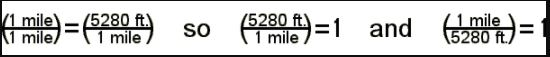We can multiply any quantity by anything equal to 1 without changing the value of the quantity. Therefore, 5280 ft/mile or 1 mile/5280 ft is a conversion factor for length. These conversion factors can change 6.20 miles to a number of meters in serial fashion thus: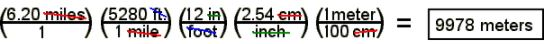Notice you can visualize the definitions you know from the units-and-definitions section in each one of the conversion factors. The definition 1 mile = 5280 ft can convert miles to feet. We want to cancel out the miles, so we place 1 mile under the 5280 ft. Now the mile units can cancel with one in the denominator and the other in the numerator. If we were to stop at this point, the answer would be in units of feet. Similarly, use 1 foot = 12 in. to go from feet to inches, placing the foot unit in the denominator to cancel with the foot unit in the numerator. The definitions and conversions in the units and measures and definitions chapter become very convenient for use as conversion factors. It is necessary to know them by rote to be able to easily use the system. If you know the definitions well, not only will you escape having to look up the right definition, but you will much more easily spot the best way to convert the numbers. The metric system definitions come from the powers of ten of the metric prefixes. The metric “staircase” method allows for changes from any magnitude to another in one conversion step by “counting the steps up or down the staircase.”

We can use the density of a material as a conversion factor between mass and volume rather than a formula in this manner: The density of mercury is 13.6 g/cc. What is the mass of two liters of mercury?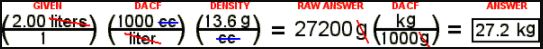This math could have been done without stopping to calculate the intermediate answer of 27200 g, but the math always works, no matter in what order it is done. In both cases we carefully accounted for the units by canceling. A stepwise orderly progression of easily remembered changes can convert units to anything you need. The metric system is particularly easy to use with DA. The definitions are multiples of ten and need scrupulous care to stay untangled. Here is a way to think of it using a change from Kilometers to millimeters. Kilometers being the larger unit, begin with one of the larger unit. The number of smaller units, (mm) is the power of ten that is the number of steps up the metric staircase. This process keeps the exponent positive.

1 km = 1x 106 mm or 1 km = E6 mm

Problems that involve many substances or a lot of addition and subtraction can become difficult with DA, but for conversions within a dimension or simple multiplication or division problems, even in serial fashion, it is a powerful tool. We can use DA when working formula problems by consistently using and canceling the appropriate units. The answer must come out in the proper dimension according to the units, or you should suspect something wrong. You may then easily convert the answer to units you want by using simple definitions as conversion factors. As you may have suspected, DA has many uses in the study of chemistry. Many common daily-living problems could be helped by thinking in DA.

## The W5P Method

One of the really difficult things to learn or teach in any science is the way to do word problems. For some students, there seems to be a mental block about it. We can show a method that may work for you, but the success of the method depends upon the background knowledge of the student in the units, dimensions, and formulas.

### W5P Method (Wild & Wonderful Way to Work Word Problems)

GIVEN – List all pertinent information with dimension symbol, number and unit.

FIND – List the dimension of the quantity requested in problem.

FORMULA – With the dimensions in GIVEN and FIND, list the formula of formulas that fit.

SOLVE – Solve the formula for what you are looking for (FIND), substitute the number values in GIVEN, and perform the math on both the units and the numbers.

ANSWER – Check the answer for likeliness, make sure the units are appropriate, express the answer in scientific notation and to the accuracy required, and draw a box around it so it is obvious which number your answer is.

Here is a sample problem using W5P method. Lead is 11.3 g/cc. What is the volume of 24.5 kg of lead? If you recognize the units of density, you can immediately spot that D = 11.3 g/cc. The symbol of the dimension, density, is D. We show the number and units of the density with the symbol. The mass is 24.5 kg. (m = 24.5 kg. ) Find the volume (V). The formula that has all the dimensions needed is the density formula from the formula list. We write it here in its original (memorized!) form. Solve for what you are looking for (volume here) using just a little bit of good old basic algebra. You would like to get the equation in the condition where you have V on one side all by itself. Do the algebra as stepwise as you need to in order to show yourself you have actually done it right. Next, substitute the measurements for the dimension symbols. Note that the kilogram and cc and gram units cancel, along with the number one thousand in the math. All we have left is to do the division and processing of the answer. The answer calls for three significant digits. The number does not need scientific notation because the number is less than one thousand and more than one thousandth.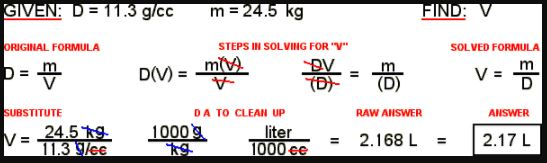Notice that the “GIVEN” section includes the symbol for the dimension of the information, the number and unit. “FIND” shows the dimension of the information requested. The formula is not labeled as such, but it is the relationship that has the requested information among the dimensions and all other dimensions in the formula are known. (Here we are looking for V and we know D and m.) The next step is to solve the formula for the dimension we need. Then substitute the given quantities for the known dimensions and process the answer.

Here is an opportunity to show you a small but useful way to save yourself some grief. Consider the unit g/cc in the above math. The unit is a fraction in the denominator. It is easier to think of as cc/g in the numerator. (The rules of math permit you to move a fraction from the denominator to the numerator if you invert the fraction.)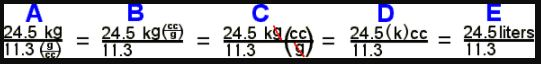Fraction A comes from the original substitution of the numbers and units into the solved equation. Fraction B shows the inversion of the fractional unit into the numerator. This is a really useful way to simplify fractions that seem complex. Fraction C shows the same fractional unit integrated into the whole fraction and cancels the unit of gram. Step C is not needed if you want to cancel the gram unit in the numerator. Fraction D shows what happens when you cancel a unit without its metric prefix. The k is just one thousand now, so k (cc) is the same as one thousand times cc which is a liter. Fraction E shows the final unit. Many students make math mistakes by failing to simplify fractions in the denominator.

## The Temperature Box

There are two types of common conversion that are not easily fit for calculation by DA because they require formulas among the different units. Temperature conversions are among the units Celsius, Kelvin, Fahrenheit, and Rankine. Acidity units are pH, hydrogen ion concentration, hydroxide ion concentration, and pOH. A conversion box is a fine teaching device for this type of unit association group.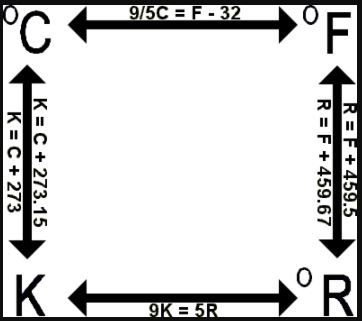(For going around the box, use K = C + 273.15 and
R = F + 459.67 to get more accurate results.)

SOME COMMON TEMPERATURES in all four scales to the nearest whole degree

 Material Point Kelvin Celsius Fahrenheit Rankine copper m.p. 1356 1083 1981 2441 gold melt pt 1336 1063 1945 2405 aluminum m.p. 933 660 1219 1680 lead melt pt 601 327 621 1081 H2O bp1atm 373 100 212 672 chicken body 313 40 104 564 human body 310 37 98.6 558 room temp. 293 20 68 528 H2O m.p. 273 0 32 492 zero °F 292 -19 0 460 mercury m.p. 234 -39 -38 422 °C = °F 233 -40 -40 420 absolute zero 0 -273 -460 0

There are only three temperature measurement scales commonly used. The Kelvin, Fahrenheit, and Celsius or Centigrade scales all have common uses. The Rankine scale is not commonly used, but it is needed here for the learning experience. Consider four identical liquid-type thermometers side-by-side with different markings on them. This is a good mental image because These four scales measure exactly the same thing. The small superscript “zero” before the symbol means “degree.” Some people make a big issue of omitting the degree sign before Kelvin. They say “Kelvins” instead of “degrees Kelvin.”

The Kelvin and Rankine scales are both absolute temperature scales. Since temperature is a measure of the average velocity of the atoms or molecules in a material, it makes sense that there is a temperature that represents the condition of no molecular motion. That temperature is called “absolute zero.” Both Kelvin and Rankine scales are absolute scales. The zero mark on these scales is at absolute zero. There are no negative temperatures on either scale. Since they have the same zero point, the relationship between them is a simple proportion. You will remember the formula as the Dog Equation – – Canine equals Arf-ive. Yes, K 9 = R 5 or, as you more commonly see it, 9 K = 5 R. No applause, please.

The size of the Celsius degree is the same as the size of the Kelvin degree. The Celsius scale just has its zero at the freezing point (or melting point, for they are the same) of water, 273.15 K. The size of the Fahrenheit degree is the same as the size of the Rankine degree. To calculate the temperature in Rankine, add 459.67 to the Fahrenheit temperature. For instance, the freezing point of water is 32 °F or 491.67 °R.

The easy way to be sure to know the conversion between Fahrenheit and Celsius is as follows: (a) Only remember one formula. (b) From the Dog Equation the proportion of Celsius and Fahrenheit is nine to five. 9/5 C must be the right proportion, and (c) Fahrenheit zero is 32 degrees below the freezing point of water. Therefore, the equation is:

9/5 C = F – 32

This is the only equation you will need or want. Some texts will ask you to memorize two equations, one solved for Fahrenheit and the other solved for Celsius. To get Celsius temperature from Fahrenheit, you must solve for ‘C,’ but, for the convenience of remembering only one easily related equation, the price is cheap.

The temperature box is a device that can give you as much practice as you need. Here is how to use it. Choose a temperature, for instance, 37 °C, normal human body temperature. Calculate around the box either clockwise or counter-clockwise. You should return to the same Celsius temperature. For rough one-time calculations, you can use the more generally quoted change between Celsius and Fahrenheit and between Fahrenheit and Rankine. For going around the temperature box, you should use the more exact numbers in the parentheses.

## Percentage Calculations

Converting raw numbers to percentages is easy once the parts are defined. A percentage is the target over the total multiplied by one hundred percent.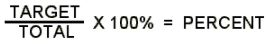There are thirty people in the classroom. Of them, seventeen are male. What percentage of males are in the classroom? ‘Seventeen males’ is the target we have defined. ‘Thirty people’ is the total. Seventeen divided by thirty times one hundred is 56.66667 to seven significant digits or 56.7 to three significant digits. Males are people, so we cancel the units. The answer is 56.7 percent.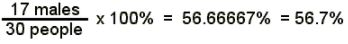In many cases, the most difficult part of using percentages is identifying the target and the total. Percentages do not have any other unit attached to them other than the percent. After dividing one unit by the same type of unit and cancelling the units, that makes sense.

## Basic Algebra

Algebra operations can be performed on equations with symbols, numbers, or measurements. An equation states that the right side of the equation is equal to the left side. The rules of manipulation of the two sides of an equation without changing the equation are: (a) you can multiply or divide both sides by the same thing, (b) you can add or subtract the same thing from both sides, (c) you can raise both sides to the same power, you can change both sides to or from any exponent. Also, you can substitute anything in an equation for something of equal value. The rules are easy, but becoming proficient takes practice. If you need the practice, there are many basic algebra textbooks and web sites. Most people need to actually DO the math in the textbook or web site rather than just reading about them.

One of the really big mistakes many novice calculators make is attempting to do too much between the ears before enough practice has been done. The best advice is that calculating with a pencil (or writing implement of any type) is much more reliable than calculating mentally. You may have heard of “back- of- the- envelope” calculations by scientists or engineers. Most people of science or technology understand that even something scratched on the back of an envelope is more reliable than mental calculation. Show your work, if not to your teacher, to yourself. Show the addition of the same thing to both sides. Show the cancellation of units. Show the stepwise conversion of what you know to what you need. Except for log and antilog work for pH calculations, the use of more complex algibraic operations in basic chemistry course is rare. The use of quadratics or complex factoring is rare.

## Direct Proportion

A boy and a girl are in the back seat of the car on a long trip through the countryside. Their mother suggests that they count the cows on their side of the car to keep them occupied. The boy sees a large number of cows on his side and begins furiously counting, “1, 2, 3, 4, 5.” It is obvious from his disappointment that he did not get a chance to count all his cows. Then a much larger herd appears on the girl’s side. She looks at the herd and proudly announces, “Six hundred and seventy seven cows.” The boy angrily says. “Oh, you couldn’t have counted that many so quickly.” She retorts, “It’s easy. All you have to do is count the legs and divide by four. Mathematically, of course, she is correct. The number of cows is proportional to the number of legs; as the number of cows increases, the number of legs increases and visa-versa. This could be expressed mathematically as: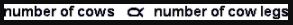You would read the proportionality as: “The number of cows is proportional to the number of cow legs.”

An example in chemistry of proportionality is the relationship of the pressure and temperature of the same gas at a constant volume. When the temperature increases, the pressure increases. When the temperature decreases, the pressure decreases. The formula for that can be expressed: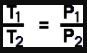## Inverse Proportion

Consider the table of hydrogen ion concentrations versus hydroxide concentrations. As the [H+] increases, the [OH-] decreases. As the [H+] decreases, the [OH-] increases. This relationship is inverse proportionality.

It is something of a joke in corporate circles that the importance of a person is inversely proportional to the number of keys carried. The CEO of the company (of ‘infinite importance’) does not have to keep any keys because all doors are opened for the CEO. The janitor (lowest in importance) has a large ring of keys. This can be shown in the proportion manner by: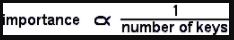This reads, “The importance is inversely proportional to the number of keys.” Using the pressure and volume of the same gas at constant temperature, inverse proportionality can be expressed mathematically by: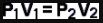## How Formulas Show Direct and Inverse Proportion

It is easy to spot proportionalities in mathematical formulas. Let’s use the universal gas law, PV = nRT, as an example. If the two variables are both in the numerator (or both in the denominator) on opposite sides of the equation, as P and T in the universal gas law, they are proportional. It two variables are on the same side of an equation, they are inversely proportional, as in P and V in the same equation.

## Problem Set #1 – Conversions Using DA

Do these conversions in the dimensional analysis style. Answer in scientific notation if > E3 or < E-3.

1. What is 1.50 mm in km?

2. How many nanoseconds are in 1.50 days?

3. How many fluid ounces (floz.) of water do you have in exactly half of a ten gallon can?

4. A car is going 60.0 MPH. How fast is that in ft/sec?

5. A car is going 62.0 MPH. How fast is that in KPH?

6. How many mm2 are there in one square kilometer?

7. How many in2 are there in an acre?

8. How many mm2 are there in 0.550 acre?

9. A car goes 0 to 60.0 MPH in 5.00 sec. Write that acceleration in m/sec2?

10. Light travels at 3.00 E8 m/sec. How fast is that in MPH?

11. An elephant has a mass of 1.80 tonnes. What is its weight in ounces?

12. Mercury has a density of 13.6 g/cc. What is that in #/gal?

13. A light year is the distance that light goes in a year. Using data from #10, how long is a light year in miles ? (Rate times time = distance)

### Answers to Problem Set #1

 1. 1.5 E-6 km 2. 1.30 E14 nsec 3. 640 Floz. 4. 88.0 ft/sec 5. 99.8 KPH 6. E12 mm2 7. 6.27E6 in2 8. 2.23 E9 mm2 9. 5.36 m/sec 10. 6.71E8 MPH 11. 6.34 E4 Oz. 12. 113 #/gal 13. 5.88 E12 mi

## Problem Set #2 – Practice With W5P Method

1. How deep would the water in a trench three inches wide and sixteen feet long have to be to contain 24.0 cubic feet of water?

2. Cloth is four feet wide as it comes on the bolt. What length of cloth would you need to cover a surface of 58.0 square yards?

3. It is 762 miles from here to Chicago. An obese chemistry teacher jogs at a rate of one mile every twenty minutes. How long would it take him to jog to Chicago if he jogged continuously and REALLY wanted to get there?

4. The density of lead is 11.4 g/cc. What is the mass of a block of lead two cm by ten cm by four cm?

5. How many liters of water could you fit into a container measuring 10.0 ft by 13.0 ft by 8.00 ft?

6. A box of qrtpsdfgh measures 24.0 x 4.00 x 12.0 inches. It has a density of 38.0 pounds per cubic foot. What is the weight of qrtpsdfgh in the box? (Notice the English system density!)

7. My basement was flooded after a big rain. The basement is rectangular 12.0 ft by 17.0 ft. The water was measured as it drained out at 51.0 cubic feet. How deep was the water in the basement?

8. A standard expressway lane is 11.0 ft wide. How long would a four lane expressway be if it had a square mile of pavement?

9. The density of gasoline is 720 g per liter. What is the mass of a jar of gasoline three cm by four cm by five cm?

10. How many liters of water could you fit into a hot tub that measures 1.50m deep and three meters in diameter? How many people? ( 1 cubic meter = 1000 L )

11. Mr. Richland has a swimming pool 10.0 ft by 20.0 ft. He had a wild party and they threw his son’s Volkswagen into the pool. The VW has a volume of 10 cubic feet. It was sealed up, but it sank because of all the lead bricks (?!?!?*?*?) in it. (a) What is the area of the pool? b) How much did the VW raise the water level?

12. How long is a string?

### Answers to Problem Set #2

 1. 72 in (6 ft) 2. 43.5 yd 3. 254 hours 4. 912 g 5. 2.94 E4 L 6. 25 1/3 # 7. 0.25 ft 8. 120 miles 9. 43.2 g 10. 1.06 E4 L 11a. 200 sq.ft. 11b. 0.6 in or 1/20 ft. 12. yes

## Problem Set #3 – W5P and DA Review Problems

1. How many ounces is 45.0 tonnes?

2. How many liters are in 8.55 cubic miles of ocean water?

3. Water has a density of one gram (1.00g) per cubic centimeter. What is that in pounds per gallon?

4. A roadsign posts a 50.0 MPH limit. What is that in KPH?

5. How large in square feet is a 525 square meter house?

6. How many square yards of paint are needed on the ceiling of a room that is 24.0 feet wide, 51.0 feet long and ten feet high?

7. Gasoline has a density of 5.83 pounds per gallon. What volume tank would you need to store three tons of gasoline?

8. The bathtub in the residential suite of the White House had to be enlarged for William Howard Taft. He weighed 370 pounds. The bathtub is four feet wide, six feet long and three feet high. How many gallons of water are needed to fill the WHT Memorial Bathtub? (1 gal = 231 cu.in.)

9. The density of water is 8.3 #/gal. What weight of water is needed to fill the WHT Memorial Bathtub?

10. Mercury is 13.6 kg/liter. What mass of mercury is needed to fill the WHT Memorial Bathtub?

11. The velocity of light is 186,000 mi/sec. It takes radio signals 17.6 minutes to go from Earth to Jupiter at their closest approach. How far apart are the two planets at that time?

12. A car takes on 22.7 L of gasoline. How many Floz is that?

13. Osmium metal is 22.0 g/mL. What’s the volume of 2.50 kg of it?

14. Concentrated sulfuric acid is 1.84 kg/L. What is the mass of 500 mL of it?

15. A man is 5 ft. 11 inches tall. How many meters is that?

16. Why is a duck?

### Answers to Problem Set #3

 1. 1.59 E6 Oz. 2. 3.56 E13 L 3. 8.30 #/gal 4. 80.5 KPH 5. 5.65 E3 ft2 6. 136 sq.yd. 7. 1.03 E3 gal 8. 539 gal 9. 4.49 E3 # 10. 2.76 E4 kg 11. 1.96 E8 mi 12. 770 Floz 13. 114 mL 14. 0.920 kg 15. 1.80 m 16. because
Scroll to Top